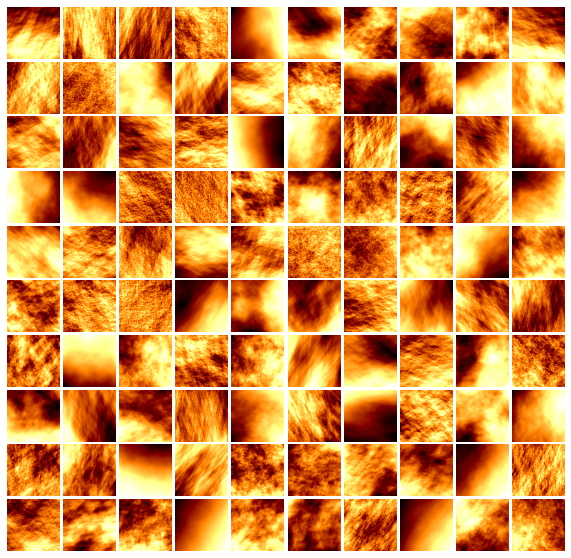# A Turning-Band Method for the Simulation of Anisotropic Fractional Brownian Fields.### Abstract

In this article, we propose a method for simulating realizations of two-dimensional anisotropic fractional Brownian fields (AFBF) introduced by Bonami and Estrade. The method is adapted from a generic simulation method called the turning-band method (TBM) due to Matheron. The TBM reduces the problem of simulating a field in two dimensions by combining independent processes simulated on oriented bands. In the AFBF context, the simulation fields are constructed by discretizing an integral equation arising from the application of the TBM to nonstationary anisotropic fields. This guarantees the convergence of simulations as the step of discretization is decreased. The construction is followed by a theoretical study of the convergence rate (the detailed proofs are available in the online supplementary materials). Another key feature of this work is the simulation of band processes. Using self-similarity properties, processes are simulated exactly on bands with a circulant embedding method, so that simulation errors are exclusively due to the field approximation. Moreover, we design a dynamic programming algorithm that selects band orientations achieving the optimal trade-off between computational cost and precision. Finally, we conduct a numerical study showing that the approximation error does not significantly depend on the regularity of the fields to be simulated, nor on their degree of anisotropy. Experiments also suggest that simulations preserve the statistical properties of the original field.

Type